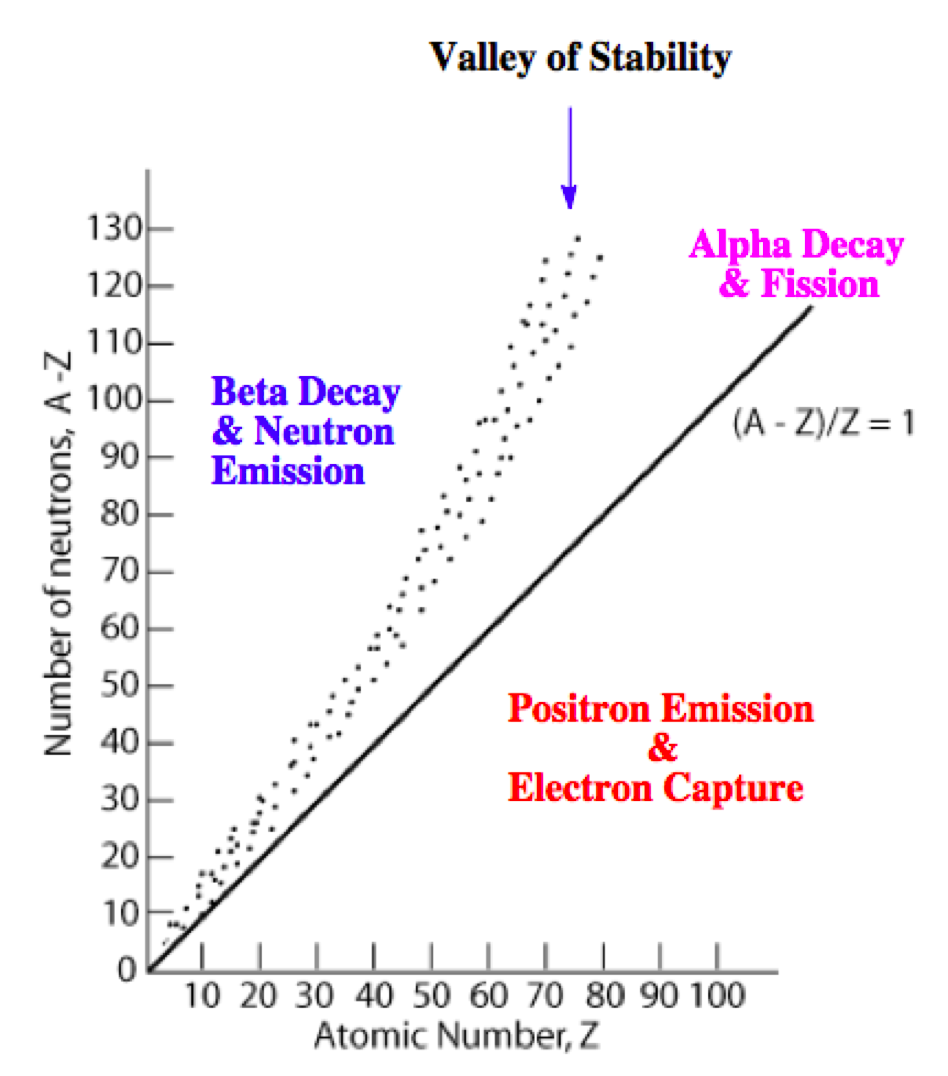# Problem: Predict the type of radioactive decay process for the following radionuclides.2968 Cu,

🤓 Based on our data, we think this question is relevant for Professor Sikora's class at NSU.

###### FREE Expert Solution

We’re being asked to predict the most likely mode of decay for 6829Cu

Recall that the stability of an isotope depends on the ratio of neutrons to protons (N/Z)

This means for stable isotopes with:

• Z ≤ 20; the N/Z ratio should be equal to 1.0

• 20 < Z ≤ 40; the N/Z ratio should be equal to 1.25

• 40 < Z ≤ 80; the N/Z ratio should be equal to 1.50

• Z < 83; the stable nuclide does not exist

The N/Z values for stable isotopes form the valley or band of stability:This means for isotopes:

• above the valley of stability: there too many neutrons in the isotope

Mode of decay: Either beta decay (0–1β) or neutron emission (10n; not in the choices)

• below the valley of stability: there too many protons in the isotope

Mode of decay: Either positron emission (01e) or electron capture (0–1e)

• with atomic mass ≥ 200 amu: there too many protons and neutrons in the isotope

Mode of decay: alpha decay (42α)